# Bihar Board Class 9th Maths Solutions Chapter 7 Triangles Ex 7.2

Bihar Board Class 9th Maths Solutions Chapter 7 Triangles Ex 7.2 Textbook Questions and Answers.

## BSEB Bihar Board Class 9th Maths Solutions Chapter 7 Triangles Ex 7.2Question 1.
In an isosceles triangle ABC, with AB = AC, the bisectors of ∠B and ∠C intersect each other at O. Join A to O. Show that:
(i) OB = OC
(ii) AO bisects ∠A.
Solution:
(i) In ∆ ABC, we haveAB = AC
⇒ ∠B = ∠C [∵ Angles opposite to equal sides are equal]
$$\frac { 1 }{ 2 }$$∠B = $$\frac { 1 }{ 2 }$$∠C
⇒ ∠OBC = ∠OCB
[∵ OB and OC bisect ∠s B and C respectively]
∴ ∠OBC = $$\frac { 1 }{ 2 }$$∠B and ∠OCB = $$\frac { 1 }{ 2 }$$∠c
⇒ OB = OC … (2)
[∵ Sides opp. to equal ∠s are equal]

(ii) Now, in ∆s ABO and ACO, we have
AB = AC [Given]
∠OBC = ∠CCB [From (1)]
∆OB = OC . [From (2)]
∴ By SAS criterion of congruence, we have
∆ABO ≅ ∆ACO
⇒ ∠BAO = ∠CAO
[∵ Corresponding parts of congruent triangles are equal]
⇒ AO bisects ∠BAC.Question 2.
In ∆ ABC, AD is the perpendicular bisector of BC (see figure). Show that ∆ ABC is an isosceles triangle in which AB = AC.Solution:
In ∆s ABD and ACD, we have
DB = DC [Given]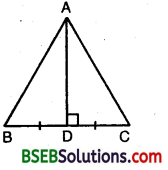∴ By SAS criterion of congruence, we have
∆ ABD ≅ ∆ ACD
⇒ AB = AC
[∵ Corresponding parts of congruent triangles are equal]
Hence, ∆ ABC is isosceles.

Question 3.
ABC is an isosceles triangle in Which altitudes BE and CF are drawn to sides AC and AB respectively (see figure). Show that these altitudes are equal.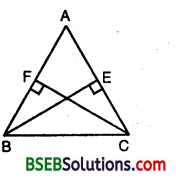Solution:
In ∆s ABE and ACF, we have
∠AEB = ∠AFC [∵ Each = 90°]
∠BAE = ∠CAF [Common]
and, BE = CF [Given]
By AAS criterion of congruence, we have
∆ ABE ≅ ∆ ACF
⇒ AB = AC
[∵ Corresponding parts of congruent triangles are equal]
Hence, ∆ ABC is isosceles.Question 4.
ABC is a triangle in which altitudes BE and CF to sides AC and AB are equal (see figure). Show that
(i) ∆ ABE ≅ ∆ ACF
(ii) AB = AC, i.e., ABC is an isosceles triangle.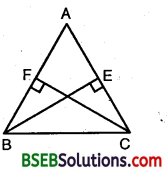Solution:
Let BE ⊥ AC and CF ⊥ AB.
In ∆s ABE and ACF, we have
∠AEB = ∠AFC [∵ Each = 90°]
∠A = ∠A [Common]
and, AB = AC [Given]
∴ By AAS criterion of congruence,
∆ ABE ≅ ∆ ACF
⇒ BE = CF
[∵ Corresponding parts of congruent triangles are equal]

Question 5.
ABC and DBC are two isosceles triangles on tile same base BC (see figure). Show that ∠ABD = ∠ACD.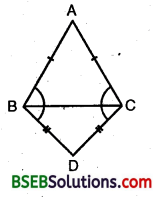Solution:
In ∆ ABC, we have
AB = AC
⇒ ∠ABC = ∠ACB
[∵ Angles opposite to equal sides are equal]
In ∆ BCD, we have
BO = CD
⇒ ∠DBC = ∠DCB … (2)
[∵ Angles opposite to equal sides are equal]
Adding (1) and (2), we have
∠ABC + ∠DBC = ∠ACB + ∠DCB
⇒ ∠ABD = ∠ACDQuestion 6.
∆ ABC is an isosceles triangle in which AB = AC. Side BA is produced to D such that AD = AB (see figure). Show that ∠BCD is a right angle.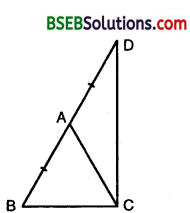Solution:
In ∆ ABC, we have
AB = AC
⇒ ∠ACB = ∠ABC … (1)
[∵ Angles opp. to equal sides are equal]
∴ AD = AC [∵AB = AC]
Thus, in ∆ ADC, we have
⇒ ∠ACD = ∠ADC … (2)
[∵ Angles opp. to equal sides are equal]
Adding (1) and (2), we get
∠ACB + ∠ACD = ∠ABC + ∠ADC
⇒ ∠BCD = ∠ABC + ∠BDC [∵ ∠ADC = ∠BDC]
⇒ ∠BCD + ∠BCD = ∠ABC + ∠BDC + ∠BCD [Adding ∠BCD on both sides]
⇒ 2∠BCD = 180°
⇒ ∠BCD = 90°
Hence, ∠BCD is a right angle.

Question 7.
ABC is a right angled triangle in which ∠A = 90 and AB = AC. Find ∠B and ∠C.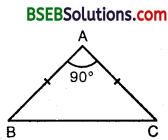Solution: We have, ∠A = 90°
AB = AC
⇒ ∠B = ∠C
[∵ Angles opp. to equal sides of a triangle are equal]
Also, ∠A + ∠B + ∠C = 180° [Angle-sum property]
⇒ 90° + 2∠B = 180° [∵ ∠C = ∠B]
⇒ 2∠B = 180° – 90° = 90°
⇒ ∠B = $$\frac { 90° }{ 2 }$$ = 45°
∴ ∠C = ∠B = 45°Question 8.
Show that the angles of an equilateral triangle are 60° each.
Solution:
Let ∆ ABC be an equilateral triangle so that AB = AC = BC.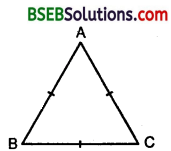Now, ∵ AB = AC
⇒ ∠B = ∠C … (1)
[∵ Angles opp. to equal sides are equal]
Also, ∵ CB = CA
⇒ ∠A = ∠B … (2)
[∵ Angles opp. to equal sides are equal]
From (1) and (2), we have
∠A = ∠B = ∠C
Also, ∠A + ∠B + ∠C = 180° [Angle-sum property]
∴ ∠A + ∠A + ∠A = 180°
⇒ 3∠A = 180° ⇒ ∠A = 60°
∴ ∠A = ∠B = ∠C = 60°
Thus, each angle of an equilateral triangle is 60°.